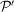International
Tables for
Crystallography
Volume A
Space-group symmetry
Edited by M. I. Aroyo

International Tables for Crystallography (2016). Vol. A, ch. 1.3, pp. 39-40

## Section 1.3.4.4.3. Crystal systems

B. Souvigniera*

aRadboud University Nijmegen, Faculty of Science, Mathematics and Computing Science, Institute for Mathematics, Astrophysics and Particle Physics, Postbus 9010, 6500 GL Nijmegen, The Netherlands
Correspondence e-mail: souvi@math.ru.nl

#### 1.3.4.4.3. Crystal systems

| top | pdf |

The point groups contained in a geometric crystal class can act on different Bravais types of lattices, which is the reason why lattice systems do not classify point groups. But the action on different types of lattices can be exploited for a classification of point groups by joining those geometric crystal classes that act on the same Bravais types of lattices. For example, the holohedryacts on primitive, face-centred and body-centred cubic lattices. The other geometric crystal classes that act on these three types of lattices are 23,, 432 and.

#### Definition

Two space groupsandwith point groupsand, respectively, belong to the same crystal system if the sets of Bravais types of lattices on whichandact coincide. Since point groups in the same geometric crystal class act on the same types of lattices, crystal systems consist of full geometric crystal classes and the point groupsandare also said to belong to the same crystal system.

Remark: In the literature there are many different notions of crystal systems. In International Tables, only the one defined above is used.

In many cases, crystal systems collect together geometric crystal classes for point groups that are in a group–subgroup relation and act on lattices with the same number of free parameters. However, this condition is not sufficient. If a point groupis a subgroup of another point group, it is clear thatacts on each lattice on whichacts. Butmay in addition act on different types of lattices on whichdoes not act.

Note that it is sufficient to consider the action on lattices with the maximal number of free parameters, since the action on these lattices implies the action on lattices with a smaller number of free parameters (corresponding to metric specializations).

#### Example

The holohedry of type 4/mmm acts on tetragonal and body-centred tetragonal lattices. The crystal system containing this holohedry thus consists of all the geometric crystal classes in which the point groups act on tetragonal and body-centred tetragonal lattices, but not on lattices with more than two free parameters. This is the case for all geometric crystal classes with point groups containing a fourfold rotation or roto­inversion and that are subgroups of a point group of type 4/mmm. This means that the crystal system containing the holohedry 4/mmm consists of the geometric classes of types 4,, 4/m, 422, 4mm,and 4/mmm.

This example is typical for the situation in three-dimensional space, since in three-dimensional space usually all the arithmetic crystal classes contained in a holohedry are Bravais arithmetic crystal classes. In this case, the geometric crystal classes in the crystal system of the holohedry are simply the classes of those subgroups of a point group in the holohedry that do not act on lattices with a larger number of free parameters.

The only exceptions from this situation are the Bravais arithmetic crystal classes for the hexagonal and rhombohedral lattices.

#### Example

A point group containing a threefold rotation but no sixfold rotation or rotoinversion acts both on a hexagonal lattice and on a rhombohedral lattice. On the other hand, point groups containing a sixfold rotation only act on a hexagonal but not on a rhombohedral lattice. The geometric crystal classes of point groups containing a threefold rotation or rotoinversion but not a sixfold rotation or rotoinversion form a crystal system which is called the trigonal crystal system. The geometric crystal classes of point groups containing a sixfold rotation or rotoinversion form a different crystal system, which is called the hexagonal crystal system.

The classification of the point-group types into crystal systems is summarized in Table 1.3.4.2.

 Table 1.3.4.2| top | pdf | Crystal systems in three-dimensional space
Crystal systemPoint-group types
Triclinic, 1
Monoclinic 2/m, m, 2
Orthorhombic mmm, mm2, 222
Tetragonal 4/mmm,, 4mm, 422, 4/m,, 4
Hexagonal 6/mmm,, 6mm, 622, 6/m,, 6
Trigonal, 3m, 32,, 3
Cubic,, 432,, 23

Remark: Crystal systems can contain at most one holohedry and in dimensions 2 and 3 it is true that every crystal system does contain a holohedry. However, this is not true in higher dimensions. The smallest counter-examples exist in dimension 5, where two (out of 59) crystal systems do not contain any holohedry.